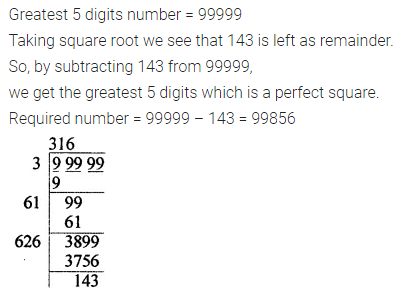# ML Aggarwal Class 8 Solutions for ICSE Maths Chapter 3 Squares and Square Roots Check Your Progress

## ML Aggarwal Class 8 Solutions for ICSE Maths Chapter 3 Squares and Square Roots Check Your Progress

Question 1.
Show that 1089 is a perfect square. Also, find the number whose square is 1089.
Solution: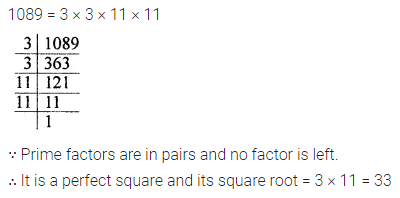Question 2.
Find the smallest number which should be multiplied by 3675 to make it a perfect square. Also, find the square root of this perfect square.
Solution: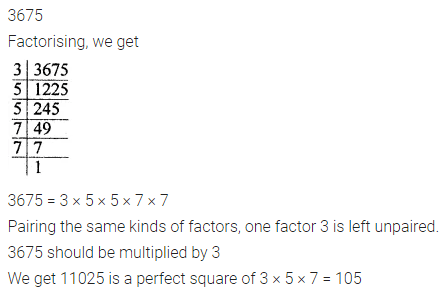Question 3.
Express 121 as the sum of 11 odd numbers.
Solution:Question 4.
How many numbers lie between 992 and 1002?
Solution:Question 5.
Write a Pythagorean triplet whose one number is 17.
Solution: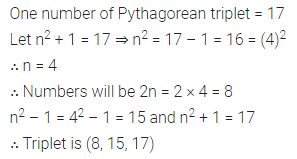Question 6.
Find the smallest square number which is divisible by each of the numbers 6, 8, 9.
Solution: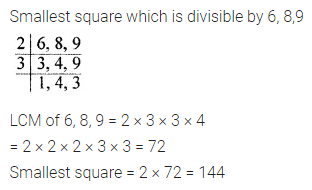Question 7.
In an auditorium, the number of rows is equal to a number of chairs in each row. If the capacity of the auditorium is 1764. Find the number of chairs in each row.
Solution: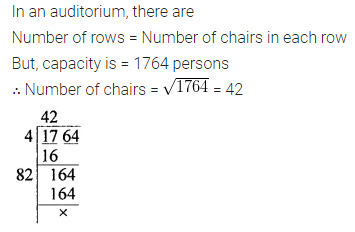Question 8.
Find the length of diagonal of a rectangle whose length and breadth are 12 m and 5 m respectively.
Solution: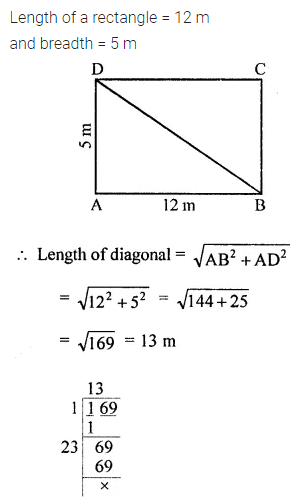Question 9.
Find the square root of 144 by successive subtraction.
Solution: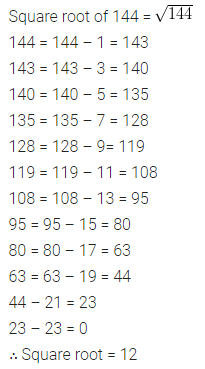Question 10.
Find the square root of the following numbers by prime factorisation:
(i) 5625
(ii) 1521
Solution:Question 11.
Find the square root of the following numbers by long division method:
(i) 21904
(ii) 108241
Solution:Question 12.
Find the square root of following decimal numbers:
(i) 17.64
(ii) 13.3225
Solution:Question 13.
Find the square root of the following fractions:Solution: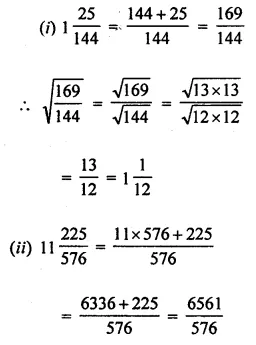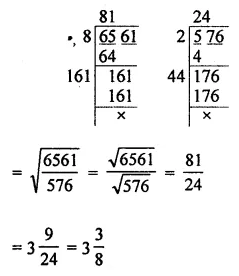Question 14.
Find the least number which must be subtracted from 2311 to make it a perfect square.
Solution:Question 15.
Find the least number which must be added to 520 to make it a perfect square.
Solution:Question 16.
Find the greatest number of 5 digits which is a perfect square.
Solution: